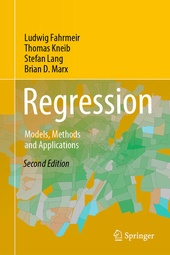# Regression

Models, Methods and Applications

Springer (Verlag)
• 2. Auflage
• |
• erscheint ca. am 24. Oktober 2021

• Buch
• |
• Hardcover
978-3-662-63881-1 (ISBN)

Now in its second edition, this textbook provides an applied and unified introduction to parametric, nonparametric and semiparametric regression that closes the gap between theory and application. The most important models and methods in regression are presented on a solid formal basis, and their appropriate application is shown through numerous examples and case studies. The most important definitions and statements are concisely summarized in boxes, and the underlying data sets and code are available online on the book's dedicated website. Availability of (user-friendly) software has been a major criterion for the methods selected and presented.

The chapters address the classical linear model and its extensions, generalized linear models, categorical regression models, mixed models, nonparametric regression, structured additive regression, quantile regression and distributional regression models. Two appendices describe the required matrix algebra, as well as elements of probability calculus and statistical inference.

In this substantially revised and updated new edition the overview on regression models has been extended, and now includes the relation between regression models and machine learning, additional details on statistical inference in structured additive regression models have been added and a completely reworked chapter augments the presentation of quantile regression with a comprehensive introduction to distributional regression models. Regularization approaches are now more extensively discussed in most chapters of the book.

The book primarily targets an audience that includes students, teachers and practitioners in social, economic, and life sciences, as well as students and teachers in statistics programs, and mathematicians and computer scientists with interests in statistical modeling and data analysis. It is written at an intermediate mathematical level and assumes only knowledge of basic probability, calculus, matrix algebra and statistics.

 Auflage: 2nd ed. 2021 Sprache: Englisch Verlagsort: Heidelberg | Deutschland Verlagsgruppe: Springer Berlin Zielgruppe: Für Beruf und Forschung Editions-Typ: Überarbeitete Ausgabe Illustrationen: Approx. 750 p. Maße: Höhe: 23.5 cm | Breite: 15.5 cm Schlagworte: ISBN-13: 978-3-662-63881-1 (9783662638811) DOI: 10.1007/978-3-662-63882-8weitere Ausgaben werden ermittelt

Ludwig Fahrmeir is Professor Emeritus at the Institute of Statistics at LMU Munich, Germany. From 1995 to 2006 he was the speaker of the Collaborative Research Center 'Statistical Analysis of Discrete Structures', supported financially by the German National Science Foundation. His main research interests include semiparametric regression, longitudinal data analysis and spatial statistics, with applications ranging from social science and risk management to public health and neuroscience.

Thomas Kneib is a Professor of Statistics at the University of Göttingen, Germany, where he is the Speaker of the interdisciplinary Centre for Statistics and Vice-Speaker of the Campus Institute Data Science. He received his PhD in Statistics at LMU Munich and, during his PostDoc phase, was Visiting Professor of Applied Statistics at the University of Ulm and Substitute Professor of Statistics at the University of Göttingen. From 2009 until 2011 he was Professor of Applied Statistics at Carl von Ossietzky University Oldenburg. His main research interests include semiparametric regression, spatial statistics and distributional regression.

Stefan Lang is a Professor of Applied Statistics at the University of Innsbruck, Austria. He received his PhD at LMU Munich. From 2005 to 2006 he was Professor of Statistics at the University of Leipzig. He is currently Associate Editor of the journal Statistical Modelling. His main research interests include semiparametric and spatial regression, multilevel modelling and complex Bayesian models, with applications, among others, in development economics, environmetrics, marketing science, real estate and actuarial science.

Brian D. Marx is Professor at the Department of Experimental Statistics at Louisiana State University, LA, USA. His main research interests include P-spline smoothing, ill-conditioned regression problems, and high-dimensional chemometric applications. He is currently serving as Coordinating Editor for the journal Statistical Modelling, is past chair of the Statistical Modelling Society, and is a Fellow of the American Statistical Association.

Introduction.- Regression Models.- The Classical Linear Model.- Extensions of the Classical Linear Model.- Generalized Linear Models.- Categorical Regression Models.- Mixed Models.- Nonparametric Regression.- Structured Additive Regression.- Distributional Regression Models.

Now in its second edition, this textbook provides an applied and unified introduction to parametric, nonparametric and semiparametric regression that closes the gap between theory and application. The most important models and methods in regression are presented on a solid formal basis, and their appropriate application is shown through numerous examples and case studies. The most important definitions and statements are concisely summarized in boxes, and the underlying data sets and code are available online on the book's dedicated website. Availability of (user-friendly) software has been a major criterion for the methods selected and presented.

The chapters address the classical linear model and its extensions, generalized linear models, categorical regression models, mixed models, nonparametric regression, structured additive regression, quantile regression and distributional regression models. Two appendices describe the required matrix algebra, as well as elements of probability calculus and statistical inference.

In this substantially revised and updated new edition the overview on regression models has been extended, and now includes the relation between regression models and machine learning, additional details on statistical inference in structured additive regression models have been added and a completely reworked chapter augments the presentation of quantile regression with a comprehensive introduction to distributional regression models. Regularization approaches are now more extensively discussed in most chapters of the book.

The book primarily targets an audience that includes students, teachers and practitioners in social, economic, and life sciences, as well as students and teachers in statistics programs, and mathematicians and computer scientists with interests in statistical modeling and data analysis. It is written at an intermediate mathematical level and assumes only knowledge of basic probability, calculus, matrix algebra and statistics.
Schweitzer Klassifikation
Thema Klassifikation
Newbooks Subjects & Qualifier
DNB DDC Sachgruppen
Dewey Decimal Classfication (DDC)
• Science
•Mathematics
•Probabilities & applied mathematics
BIC Classifikation
BISAC Classifikation
Warengruppensystematik 2.0

Noch nicht erschienen

 160,49 € inkl. 7% MwSt.